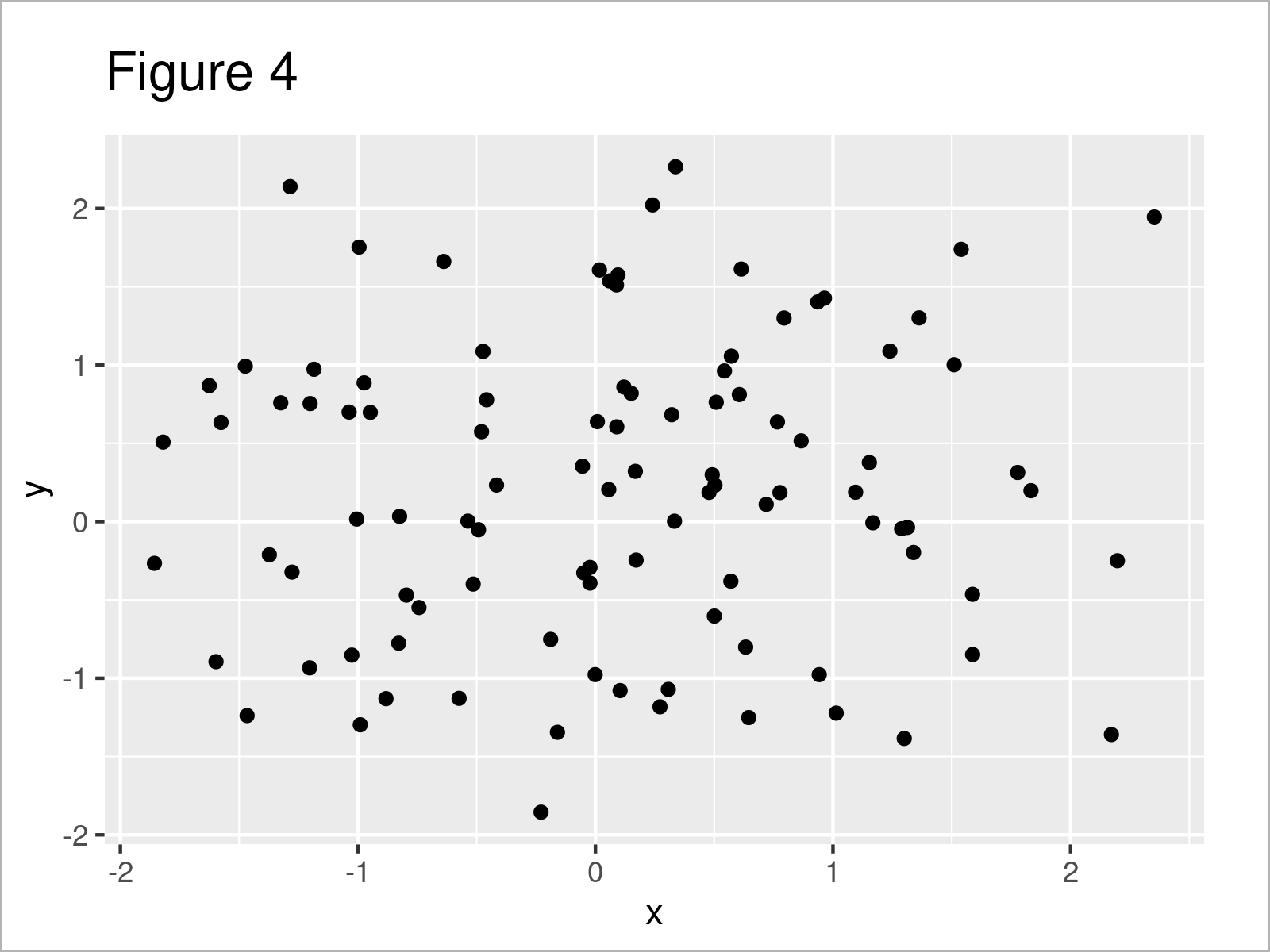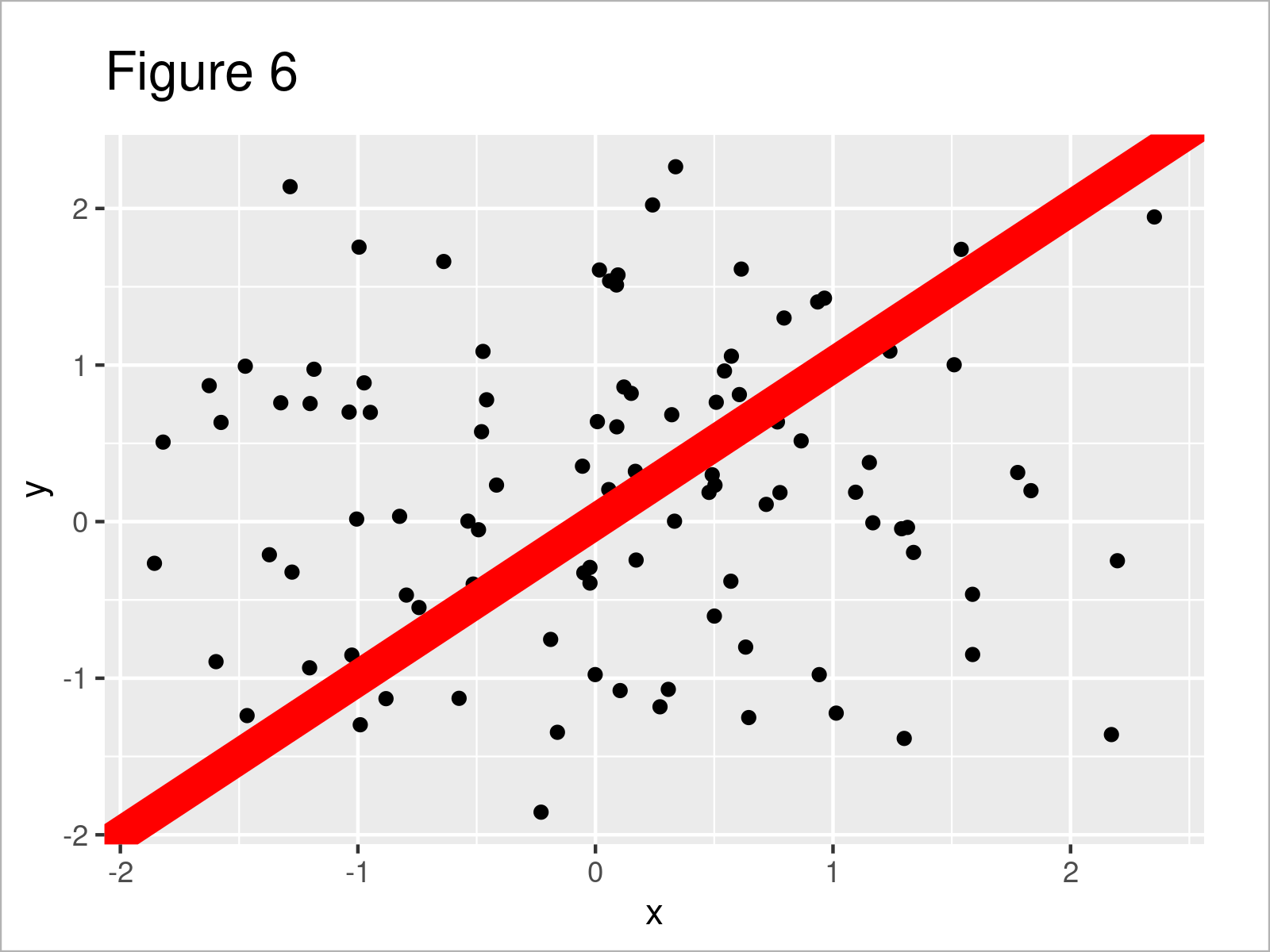# Add Diagonal Line to Plot in R (2 Examples)

In this tutorial, I’ll illustrate how to draw a diagonal line to a plot in R.

Let’s do this:

## Example Data

Consider the following example data:

```set.seed(3256733)                   # Create example data frame
data <- data.frame(x = rnorm(100),
y = rnorm(100))Table 1 shows the first six data points of our example data – as you can see, our data consists of two numerical columns.

## Example 1: Draw Diagonal Line to Base R Plot

Example 1 illustrates how to overlay a diagonal line on top of a Base R plot.

Let’s first create a graphic without any lines:

`plot(data)                          # Draw data without line`The output of the previous R programming syntax is shown in Figure 1 – A Base R scatterplot.

Next, we can add a diagonal line to this plot using the abline function and the coef argument:

```plot(data)                          # Draw data with line
abline(coef = c(0, 1))```By executing the previous R programming syntax, we have plotted Figure 2, i.e. a Base R scatterplot with a thin black diagonal line.

In the next step, we may specify some further arguments within the abline function to adjust the design of our line. The following code displays our line in red and thicker than before:

```plot(data)                          # Change color & thickness of line
abline(coef = c(0, 1),
col = "red",
lwd = 5)```Looks good!

## Example 2: Draw Diagonal Line to ggplot2 Plot

This example explains how to use the ggplot2 package to draw a plot with a diagonal line.

We first need to install and load the ggplot2 package to RStudio:

```install.packages("ggplot2")         # Install ggplot2 package

In the next step, we can draw a ggplot2 scatterplot with default settings (i.e. without a line):

```ggp <- ggplot(data, aes(x, y)) +    # Draw data without line
geom_point()
ggp```The output of the previously shown code is illustrated in Figure 4 – A ggplot2 scatterplot of our example data.

In the next step, we may use the geom_abline function as well as the intercept and slope arguments to add a diagonal line:

```ggp +                               # Draw data with line
geom_abline(intercept = 0,
slope = 1)```The output of the previous R programming syntax is shown in Figure 5 – A ggplot2 scatterplot with diagonal line.

In addition, we might use the col and size arguments to change the color and thickness of our line:

```ggp +                               # Change color & thickness of line
geom_abline(intercept = 0,
slope = 1,
col = "red",
size = 5)```By running the previous R programming code, we have drawn Figure 6, i.e. a ggplot2 graph with thick and red diagonal line.

## Video & Further Resources

In case you need further information on the R programming code of this article, you may want to have a look at the following video on my YouTube channel. In the video, I explain the contents of this tutorial:

Please accept YouTube cookies to play this video. By accepting you will be accessing content from YouTube, a service provided by an external third party.If you accept this notice, your choice will be saved and the page will refresh.

In addition, you might want to read the related tutorials on my website. A selection of tutorials is listed below:

At this point you should know how to add a diagonal line to a graph in the R programming language. Don’t hesitate to let me know in the comments below, if you have additional questions.

Subscribe to the Statistics Globe Newsletter